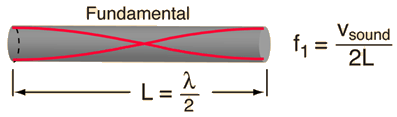# Open Air Column Frequency

At temperature C = F,

the speed of sound is m/s = ft/s = mi/hr.

An open cylindrical air column 0f length L = m = ft

will produce a fundamental frequency:= Hz

In actual practice, the position of the antinode is slightly outside the open end, and an end correction of about 0.6 times the radius of the pipe should be added to each end to get the effective acoustic length.

 Discussion of open column Calculation for closed column
Index

Wave concepts

Resonance concepts

 HyperPhysics***** Sound R Nave
Go Back